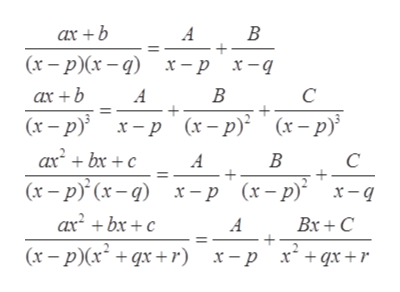# How do you complete a partial fraction decomposition? Specifically, when a binomial is over a quadratic?

Question
6 views

How do you complete a partial fraction decomposition? Specifically, when a binomial is over a quadratic?

check_circle

star
star
star
star
star
1 Rating
Step 1

Let an example to understand partial fraction decomposition:

Step 2

If numerator have maximum power equal or more than denominator then numerator is divided

by the denominator before writing its decomposition form.

Partial fraction decomposition formulas:help_outlineImage TranscriptioncloseВ ax +b A (х — р)(х — q) х-р х-д В C ax +b A (x-p x-p (x-p) axbxc (x-p (x-) x-p (x-p) x-q (x-p В C A axbxc Bx+C A (x-p(xqx+r) x + qx +r х — р fullscreen
Step 3

Solving the above...

### Want to see the full answer?

See Solution

#### Want to see this answer and more?

Solutions are written by subject experts who are available 24/7. Questions are typically answered within 1 hour.*

See Solution
*Response times may vary by subject and question.
Tagged in

### Algebra